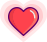## 不再惧怕！二叉树结构相关算法总结 | 原力计划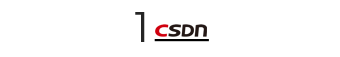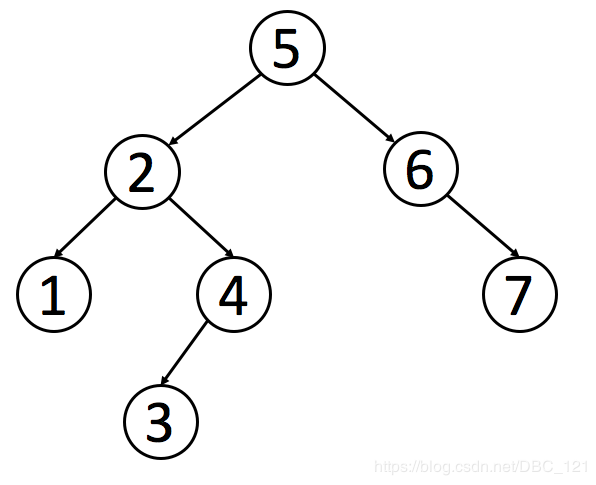``````1public class TreeNode {
2      int val;
3      TreeNode left;
4      TreeNode right;
5      TreeNode(int x) { val = x; }
6}
``````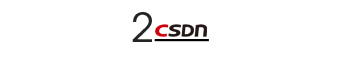``````1public void preorderTraverse(TreeNode root){
2    System.out.print(node.val+" ");
3    preorderTraverse(root.left);
4    preorderTraverse(root.right);
5}
``````

``````1public void inorderTraverse(TreeNode root){
2    inorderTraverse(root.left);
3    System.out.print(node.val+" ");
4    inorderTraverse(root.right);
5}
``````

``````1public void postorderTraverse(TreeNode root){
2    postorderTraverse(root.left);
3    postorderTraverse(root.right);
4    System.out.print(node.val+" ");
5}
``````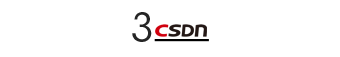`````` 1public List<Integer> preorderTraversal(TreeNode root) {
2    List<Integer> list = new ArrayList<>();
3    if(root==null){
4        return list;
5    }
6    Stack<TreeNode> stack = new Stack<>();
7    stack.push(root);
8    while(!stack.isEmpty()){
9        TreeNode res = stack.pop();
10        if(res.right != null)
11            stack.push(res.right);
12        if(res.left != null)
13            stack.push(res.left);
15
16    }
17    return list;
18}
``````

`````` 1public List<Integer> inorderTraversal(TreeNode root) {
2    List<Integer> list = new ArrayList<>();
3    if(root==null){
4        return list;
5    }
6    Stack<TreeNode> stack = new Stack<>();
7    TreeNode curr = root;
8    while(curr != null || !(stack.isEmpty())){
9        if(curr!= null){
10            stack.push(curr);
11            curr = curr.left;
12        }else{
13            curr = stack.pop();
15            curr = curr.right;
16        }
17    }
18    return list;
19}
``````

`````` 1public List<Integer> preorderTraversal(TreeNode root) {
2    List<Integer> list = new ArrayList<>();
3    if(root==null){
4        return list;
5    }
6    Stack<TreeNode> stack = new Stack<>();
7    stack.push(root);
8    while(!stack.isEmpty()){
9        TreeNode res = stack.pop();
10        if(res.left != null)
11            stack.push(res.left);
12        if(res.right != null)
13            stack.push(res.right);
15
16    }
17    list.reserve();
18    return list;
19}
``````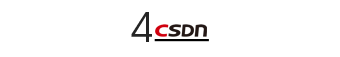*二叉树的最大深度

``````1public int maxDepth(TreeNode root) {
2    if(root==null){
3        return 0;
4    }
5    int left = maxDepth(root.left);
6    int right = maxDepth(root.right);
7    return Math.max(left,right)+1;
8}
``````

*二叉树的镜像

`````` 1public void Mirror(TreeNode root) {
2    if(root!=null){
3        if(root.left!=null || root.right!= null){
4            TreeNode temp =root.left;
5            root.left=root.right;
6            root.right=temp;
7        }
8        Mirror(root.left);
9        Mirror(root.right);
10    }
11
12}
``````

*对称二叉树

`````` 1boolean isSymmetrical(TreeNode pRoot){
2    if(pRoot == null)
3        return true;
4    return real(pRoot.left,pRoot.right);
5}
6public boolean real(TreeNode root1,TreeNode root2){
7    if(root1 == null && root2 == null){
8        return true;
9    }
10    if(root1 ==null || root2 == null){
11        return false;
12    }
13    if(root1.val != root2.val){
14        return false;
15    }
16    return real(root1.left,root2.right)&&real(root1.right,root2.left);
17}
``````

*路径总和

`````` 1public class Solution {
2    private ArrayList<Integer> list = new ArrayList<Integer>();
3    private ArrayList<ArrayList<Integer>> listAll = new ArrayList<ArrayList<Integer>>();
4    public ArrayList<ArrayList<Integer>> FindPath(TreeNode root,int target) {
5        if(root == null)
6            return listAll;
8        target -= root.val;
9        if(target == 0 && root.left==null && root.right == null){
11        }
12        FindPath(root.left,target);
13        FindPath(root.right,target);
14        list.remove(list.size()-1);
15       return listAll;
16    }
17}
``````

*重建二叉树

`````` 1 public TreeNode reConstructBinaryTree(int [] pre,int [] in) {
2    return reConstructBinaryTree(pre,0,pre.length-1,in,0,in.length-1);
3}
4public TreeNode reConstructBinaryTree(int [] pre,int startpre,int endpre,int [] in,int startin,int endin){
5    if(startpre > endpre || startin > endin){
6        return null;
7    }
8    TreeNode root = new TreeNode(pre[startpre]);
9    for(int i =startin;i<=endin;i++){
10        if(in[i] == pre[startpre]){
11            root.left = reConstructBinaryTree(pre,startpre+1,startpre+i-startin,in,startin,i-1);
12            root.right = reConstructBinaryTree(pre,startpre+i-startin+1,endpre,in,i+1,endin);
13        }
14    }
15    return root;
16}
``````

*二叉搜索树的最近公共祖先

`````` 1class Solution {
2    public TreeNode lowestCommonAncestor(TreeNode root, TreeNode p, TreeNode q) {
3        if(root == null || root == p || root == q){
4            return root;
5        }
6        TreeNode left = lowestCommonAncestor(root.left,p,q);
7        TreeNode right = lowestCommonAncestor(root.right,p,q);
8        if(left!=null && right!=null){
9            return root;
10        }
11        return left!=null?left:right;
12    }
13}
``````

*二叉树的序列化和反序列化

`````` 1序列化：
2public String serialize(TreeNode root) {
3    if (root == null) {
4        return null;
5    }
6    // 利用二叉树的层次遍历方式进行序列化
7    StringBuilder res = new StringBuilder();
10    while (!queue.isEmpty()) {
11        TreeNode node = queue.remove();
12        if (node != null) {
13            res.append(node.val).append(",");
16        } else {
17            res.append("null,");
18        }
19    }
20    return res.toString();
21}
22反序列化：
23public TreeNode deserialize(String data) {
24    if (data == null || data.length() == 0) {
25        return null;
26    }
27    String[] dataArr = data.split(",");
28    // 层次遍历逆向还原二叉树
29    int index = 0;
30    TreeNode root = toNode(dataArr[index]);
33    while (index < dataArr.length - 2 && !queue.isEmpty()) {
34        TreeNode cur = queue.remove();
35        // 添加左子节点
36        TreeNode leftNode = toNode(dataArr[++index]);
37        cur.left = leftNode;
38        // 队列中的节点用于为其赋值孩子节点，若该节点本身为 null，
39        // 没有孩子节点，便不再添加到队列中，下同理
40        if (leftNode != null) {
42        }
43        // 添加右子节点
44        TreeNode rightNode = toNode(dataArr[++index]);
45        cur.right = rightNode;
46        if (rightNode != null) {
48        }
49    }
50    return root;
51}
52
53private TreeNode toNode(String val) {
54    if (!"null".equals(val)) {
55        return new TreeNode(Integer.parseInt(val));
56    } else {
57        return null;
58    }
59}
``````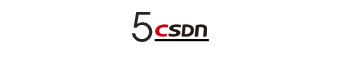• 首先将根节点放入队列中。

• 从队列中取出第一个节点，并检验它是否为目标。

• 如果找到目标，则结束搜索并回传结果。

• 否则将它所有尚未检验过的直接子节点加入队列中。

• 若队列为空，表示整张图都检查过了——亦即图中没有欲搜索的目标。结束搜索并回传“找不到目标”。

• 重复步骤2。

`````` 1public List<List<Integer>> levelOrder(TreeNode root) {
2    List<List<Integer>> res = new ArrayList<List<Integer>>();
3    List<TreeNode> quene = new ArrayList<TreeNode>();
4    if(root == null){
5        return res;
6    }
8    while(quene.size()!=0){
9        int count = quene.size();
10        List<Integer> list = new ArrayList<Integer>();
11        while(count>0){
12            TreeNode temp =quene.remove(0);
14            if(temp.left!=null){
16            }
17            if(temp.right!=null){
19            }
20            count--;
21        }
23    }
24    return res;
25}
``````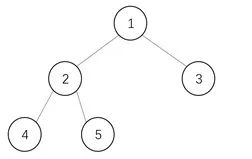• 1有左孩子2，将1放入栈中，移动到节点2；

• 2有左孩子4，将2放入栈中，移动到节点4；

• 4左孩子为空，输出节点4，此时节点4右孩子也为空，弹栈回到节点2；

• 输出节点2，节点2有右孩子5，移动到节点5；

• 5左孩子为空，输出节点5，此时节点5右孩子也为空，弹栈回到节点1；

• 设置节点1为Current节点；

• Current节点移动到左孩子节点2，并删除父节点的左指针，使其指向为null，即删除erase1；

• Current节点移动到左孩子节点4，并删除父节点的左指针，使其指向为null，即删除erase2；

• 节点4左孩子为空，输出节点4，移动到右孩子节点2；

• 节点2无左孩子(指针指向null)，输出节点2，移动到右孩子节点5；

• 节点5无左孩子，输出节点5，移动到右孩子节点1；

• 节点2无左孩子(指针指向null)，输出节点1，移动到右孩子节点3；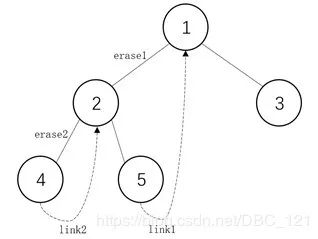`````` 1void Morris_inorderTraversal(TreeNode root) {
2    TreeNode curr = root;
3    TreeNode pre;
4    while (curr != null) {
5        if (curr.left == null) {    // 左孩子为空
6            System.out.print(curr.val+" ");
7            curr = curr.right;
8        }
9        else {   // 左孩子不为空
10            // 找左子树中的最右节点
11            pre = curr.left;
12            while (pre.right != null) {
13                pre = pre.right;
14            }
15            // 删除左孩子，防止循环
16            pre.right = curr;
17            TreeNode temp = curr;
18            curr = curr.left;
19            temp.left = null;
20        }
21    }
22}
``````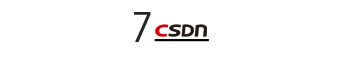AVL树

AVL 树是一种平衡二叉树，平衡二叉树递归定义如下：

• 左右子树的高度差小于等于 1。

• 其每一个子树均为平衡二叉树。

• 平衡因子：某个结点的左子树的高度减去右子树的高度得到的差值。

• AVL 树：所有结点的平衡因子的绝对值都不超过 1 的二叉树。

``````1public class TreeNode {
2      int val;
3      int height;
4      TreeNode left;
5      TreeNode right;
6      TreeNode(int x) { val = x; }
7}
``````

• 在插入结点时， 沿插入的路径更新结点的高度值

• 在删除结点时（delete），沿删除的路径更新结点的高度值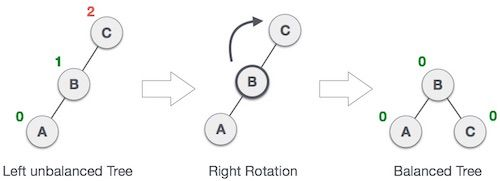`````` 1TreeNode treeRotateRight(TreeNode root) {
2    TreeNode left = root.left;
3
4    root.left = left.right; // 将将要被抛弃的节点连接为旋转后的 root 的左孩子
5    left.right = root; // 调换父子关系
6
7    left.height = Math.max(treeHeight(left.left), treeHeight(left.right))+1;
8    right.height = Math.max(treeHeight(right.left), treeHeight(right.right))+1;
9
10    return left;
11}
``````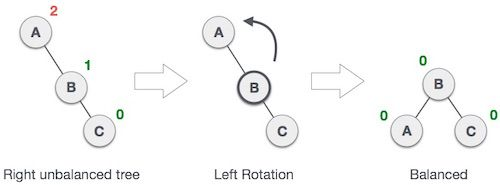`````` 1TreeNode treeRotateLeft(TreeNode root) {
2    TreeNode right = root.ight;
3
4    root.right = right.left;
5    right.left = root;
6
7    left.height = Math.max(treeHeight(left.left), treeHeight(left.right))+1;
8    right->height = Math.max(treeHeight(right.left), treeHeight(right.right))+1;
9
10    return right;
11}
``````

• LL 型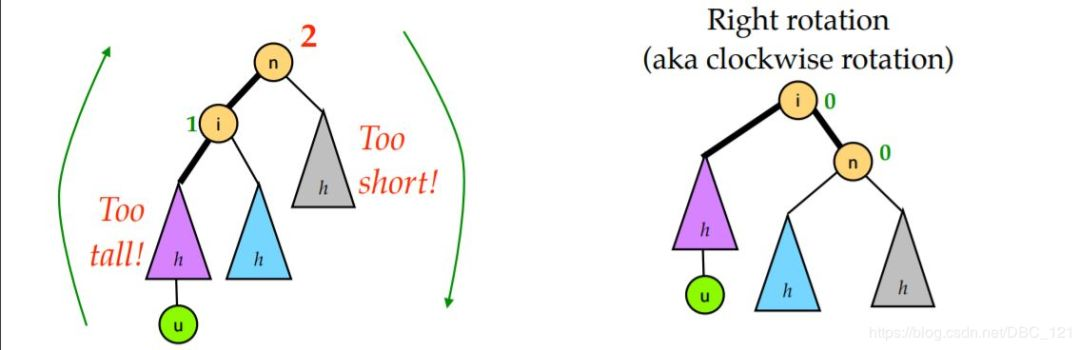• RR 型RR 型的情况和 LL 型完全对称。只需要对节点 n 进行一次左旋即可修正。

• LR 型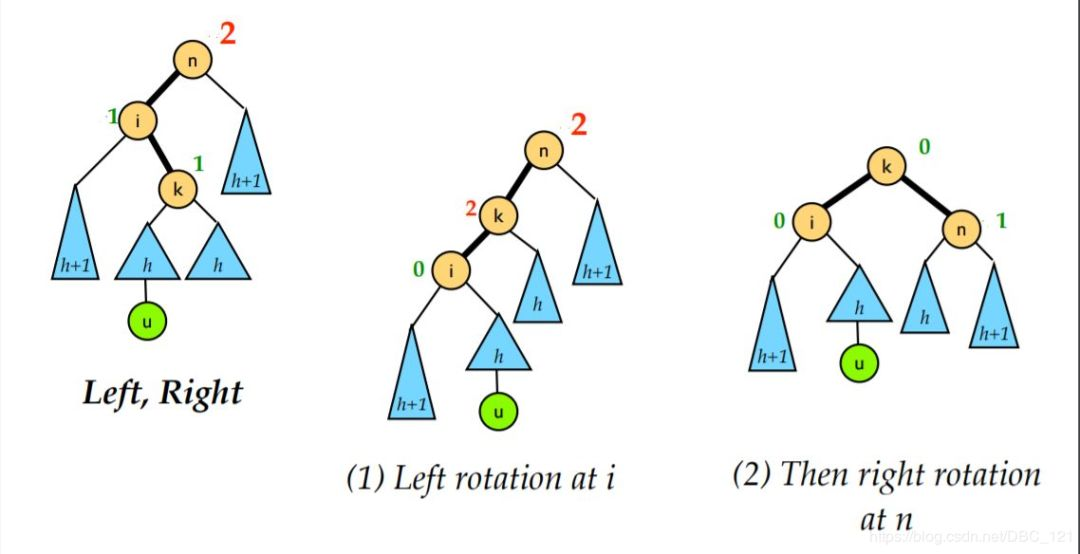LR 就是将新的节点插入到了 n 的左孩子的右子树上导致的不平衡的情况。这时我们需要的是先对 i 进行一次左旋再对 n 进行一次右旋。

• RL 型

RL 就是将新的节点插入到了 n 的右孩子的左子树上导致的不平衡的情况。这时我们需要的是先对 i 进行一次右旋再对 n 进行一次左旋。

`````` 1int treeGetBalanceFactor(TreeNode root) {
2    if(root == NULL)
3        return 0;
4    else
5        return x.left.height - x.right.height;
6}
7
8TreeNode treeRebalance(TreeNode root) {
9    int factor = treeGetBalanceFactor(root);
10    if(factor > 1 && treeGetBalanceFactor(root.left) > 0) // LL
11        return treeRotateRight(root);
12    else if(factor > 1 && treeGetBalanceFactor(root.left) <= 0) { //LR
13        root.left = treeRotateLeft(root.left);
14        return treeRotateRight(temp);
15    } else if(factor < -1 && treeGetBalanceFactor(root.right) <= 0) // RR
16        return treeRotateLeft(root);
17    else if((factor < -1 && treeGetBalanceFactor(root.right) > 0) { // RL
18        root.right = treeRotateRight(root.right);
19        return treeRotateLeft(root);
20    } else { // Nothing happened.
21        return root;
22    }
23}
``````

https://blog.csdn.net/DBC_121/article/details/104584060

【End】